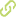# Approximate solutions of delay differential equations with constant and variable coefficients by the enhanced multistage homotopy perturbation method•
• Overview
•
• Identity
•
•
• View All
•

### Abstract

• © 2015 D. Olvera et al.We expand the application of the enhanced multistage homotopy perturbation method (EMHPM) to solve delay differential equations (DDEs) with constant and variable coefficients. This EMHPM is based on a sequence of subintervals that provide approximate solutions that require less CPU time than those computed from the dde23 MATLAB numerical integration algorithm solutions. To address the accuracy of our proposed approach, we examine the solutions of several DDEs having constant and variable coefficients, finding predictions with a good match relative to the corresponding numerical integration solutions.

### Publication date

• January 1, 2015